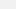# How to Calculate Your Weight On the Habitable Mega-Earth

## A back-of-the-napkin calculation.

|
Jun 3 2014, 7:05pm##### Image: Harvard University

Yesterday, scientists at Harvard University announced the discovery of a new class of planet—the "mega Earth," a rocky, potentially habitable planet that is just twice the size of Earth but has a mass of more than 17 times our planet. This news spurred two popular questions on Facebook: "When can we go there?" and "How much would you weigh on the 'Godzilla of Earths?'"Well, I can't answer the first question for sure, but I feel pretty confident in saying that it won't be in our lifetimes. And, sorry to break it to you, I can't answer the second question either—I don't know how much you weigh, dude. But I can tell you how much Steve would weigh on the planet, and I can teach you how to calculate how much you would weigh, too.

Here at Motherboard, we have a long tradition of back-of-the-napkin calculations that dates back at least one week, and a long tradition of reader-writer engagement, too. And, it turns out that calculating your weight on another planet isn't too hard, as long as you know its mass and radius. Luckily, the researchers, using the Kepler Space Telescope, were able to estimate both of those things: Kepler-10c's radius is 2.35 times that of Earth, and its mass is 17.2 times that of Earth.

One of the fundamentally mind-blowing factoids you learn in any 5th grade physics class (we're still just calling it "science" at that age, right?) is that weight and mass aren't the same thing—and that you would weigh something completely different on another planet. How much those numbers differ (compared to your weight on Earth), is directly related to a planet's surface gravity, which can be calculated with a handy formula developed, perhaps unsurprisingly, by the father of gravity, our good friend Sir Isaac Newton. It looks like this:See there? I literally used a napkin, and I'm going to show my work, except for the work done by Google's scientific calculator, which was indispensable in this endeavor.

In this formula, g is a planet's surface gravity, the big G is what is known as the gravitational constant, which, to be totally honest, I don't understand all that well but can be referred to for our purposes as a number that makes this whole thing work. Newton figured that number out as well. That number is, roughly, 6.67 times 10 to the -11th power. M is mass in kilograms, and r is the planet's radius in meters.

As is standard in these kinds of studies, Kepler-10c's vital stats were expressed in terms of Earth, so we don't have numbers we can just plug into this equation—we have to convert them into standard units before we can do any calculations. Or, we could do a logarithmic function to just compare them directly, but I skipped a lot of pre calculus classes and don't even remember what a logarithmic function is. So we're gonna do this the old-fashioned way. Also, quick shoutout to the users of the Ask Math subreddit, who helped me out when a misplaced decimal was screwing with my answer. Don't misplace your decimals, folks.

Earth's mass is 5.98 & 10 to the 24th power kilograms, give or take some amount of kilograms that doesn't really matter for our purposes. Its radius is 6378 kilometers, which seems kinda small, doesn't it? The equation won't work unless we convert that to meters, so we're left with 6.378 times 10 to the 6th power meters.

That leaves us with this:If we multiply that out, we find that the Godzilla planet's mass is 1.02856 times 10 to the 26th power kilograms, and its radius is 2.2464914 times 10 to the 14th power meters. Let's plug those into the equation:Multiply that out a bit, and you're left with this mess:Which simplifies down to a much more manageable 30.53 meters per second squared. That's the surface gravity of the Godzilla planet. That means, if the Godzilla-civilization's presumable Godzilla monsters picked you up and dropped you off the top of its version of Tokyo, you would accelerate towards its rocky ground at a speed of 30.53 meters per second, squared. Which is more than three times as fast as you'd fall on Earth. It'd probably hurt about the same, because the human body is a feeble thing.

Anyways, we're not done yet—if you were to land on a pile of alien pillows and survived, how much would you, I mean, Steve, weigh? Well, gravity here on Earth is roughly 9.81 meters per second squared, so we can calculate exactly how much more the gravity is on Kepler-10c with this equation.

Steve weighs 200 pounds on Earth (really, Steve? Such an even number). Now, all we have to do is this:So, Steve would weigh in at about 622 pounds. Which means he'd look like this:Just kidding—his mass would stay the same. Haven't you been paying any attention? So—if you want to calculate your own weight on the Godzilla planet, all you have to do is take your weight and multiply it by 3.1, which is way easier than messing with the whole formula. But now you know how to do it.By the way, for haters like Mitch who have suggested that the planet's gravity would crush you: Humans can withstand sustained exposure to roughly 5 g, so while you probably couldn't dunk a basketball on Kepler-10c, your bones wouldn't immediately crumble into nothingness, either. Those of you who suggested we'd melt have a better argument: Kepler-10c regularly sees temperatures of up to 591 degrees Fahrenheit. Bring an air conditioner, but not your powered exoskeleton.